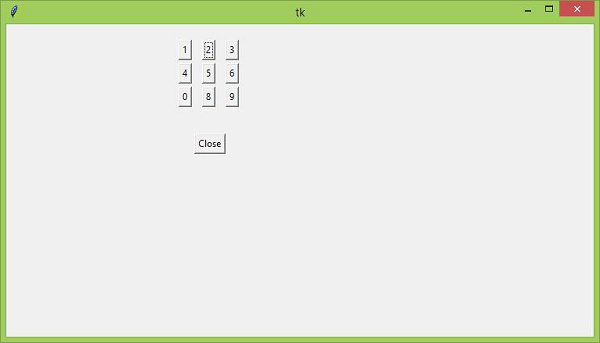# Setting the focus to a specific Tkinter entry widget

Tkinter has many universal methods that add functionalities to widgets and elements. In order to set the focus to a particular widget, we have the focus_set() method which is used to focus a specific widget in a group of widgets present in an application.

## Example

In this example, we have created numeric keys in the range 0-9. We have set the focus for Numeric key ‘2’.

#Import the required libraries
from tkinter import *
import webbrowser
#Create an instance of tkinter frame
win = Tk()
win.geometry("750x400")
#Define function for different operations
def close():
win.destroy()
#Create a Label widget
text=Label(win, text="", font=('Helvetica bold ',25))
text.place(x= 110, y= 50,)
#Create Button widgets
b1=Button(win, text= "1")
b1.place(x=220, y=20)
b2=Button(win, text= "2")
b2.place(x=250, y=20)
b3=Button(win, text= "3")
b3.place(x=280, y=20)
b4=Button(win, text= "4")
b4.place(x=220, y=50)
b5=Button(win, text= "5")
b5.place(x=250, y=50)
b6=Button(win, text= "6")
b6.place(x=280, y=50)
b7=Button(win, text= "7")
b7.place(x=280, y=80)
b8=Button(win, text= "8")
b8.place(x=250, y=80)
b9=Button(win, text= "9")
b9.place(x=280, y=80)
b0=Button(win, text= "0")
b0.place(x=220, y=80)
#Set the focus on button b1
b2.focus_set()
b2=Button(win, text= "Close", command= close)
b2.place(x=240,y= 140)
win.mainloop()

## Output

Running the above code will display the focus for numeric key ‘2’.To change the focus of the widget, just add the focus_set.() method to the widget you want to set focus on.